# Tensorflow Library

## A brief Introduction

Tensorflow is a deep learning library that makes building and deploying Deep Learning Applications super easy. If you wondered what this library is all about wait no more, keep reading the blog to find what makes Tensorflow unique.

This blog provides an overview to the Tensorflow library and provides a brief introduction to the topic with some important keywords, it’s installation and demo code.

# What is TensorFlow ?

• It is a free and open-source platform for high-performance numerical computation, specifically for ML and Deep Learning.
• Has a flexible architecture and can be deployed across a variety of platforms (CPUs, GPUs and TPUs) as well…

# Introduction to Docker

## Learn the why, what and how of Docker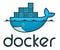Image credits: flickr.com

In this blog we’ll try to understand one of the most popular tools used to containerize and deploy applications over the internet i.e. Docker. It makes deploying applications extremely simple.

We will try to look at the things that make Docker so special and learn how you can build, deploy, and fetch applications using Docker & Docker Hub using just a few steps.

# What is Docker ?

• It is a tool used to create, deploy and run applications by using containers.
• Containers allows developers to package up an application with all the parts it needs.
• Containers are isolated from one another and bundle their…

# Introduction

Support Vector Machines (SVMs) are a set of supervised learning methods which learn from the dataset and can be used for both regression and classification. An SVM is a kind of large-margin classifier: it is a vector space based machine learning method where the goal is to find a decision boundary between two classes that is maximally far from any point in the training data.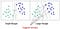Source : Towards Data Science

# Support Vectors :

The term Support Vectors refers to the co-ordinates of individual observation. Support Vector Machine is a frontier which best segregates the two classes using a hyperplane/ line.

# Random Forest Classification

## and its implementation in Python

In this blog we’ll try to dig deeper into Random Forest Classification. Here we will learn about ensemble learning and will try to implement it using Python.

You can find the code over here.

# Random Forest Classifier :

It is an ensemble tree-based learning algorithm. The Random Forest Classifier is a set of decision trees from randomly selected subset of training set. It aggregates the votes from different decision trees to decide the final class of the test object.

# Ensemble Algorithm :

Ensemble algorithms are those which combines more than one algorithms of same or different kind for classifying objects. …

# Introduction

Logistic Regression is a Supervised learning algorithm widely used for classification. It is used to predict a binary outcome (1/ 0, Yes/ No, True/ False) given a set of independent variables. To represent binary/ categorical outcome, we use dummy variables.

Logistic regression uses an equation as the representation, very much like linear regression. It is not much different from linear regression, except that a Sigmoid function is being fit in the equation of linear regression.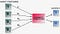Logistic Regression

Simple Linear and Multiple Linear Regression Equation:

`y = b0 + b1x1 + b2x2 + ... + e`

Sigmoid function :

`p = 1 /…`

# Decision Tree Classification

## An introduction to Decision Tree Classifier

A Decision Tree is a simple representation for classifying examples. It is a Supervised Machine Learning where the data is continuously split according to a certain parameter.

1. Nodes : Test for the value of a certain attribute.
2. Edges/ Branch : Correspond to the outcome of a test and connect to the next node or leaf.
3. Leaf nodes : Terminal nodes that predict the outcome (represent class labels or class distribution).

# K-Nearest Neighbors (KNN) Algorithm

## A Brief Introduction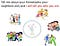Simple Analogy for K-Nearest Neighbors (K-NN)

In this blog, we’ll talk about one of the most widely used machine learning algorithms for classification, which is the K-Nearest Neighbors (KNN) algorithm. K-Nearest Neighbor (K-NN) is a simple, easy to understand, versatile and one of the topmost machine learning algorithms that find its applications in a variety of fields.

In this blog we’ll try to understand what is KNN, how it works, some common distance metrics used in KNN, its advantages & disadvantages along with some of its modern applications.

# What is K-NN ?

K-NN is a non-parametric and lazy learning algorithm. Non-parametric means there is no assumption for underlying data distribution…

# Random Forest Regression

## Along with its implementation in Python

In this blog we’ll try to understand one of the most important algorithms in machine learning i.e. Random Forest Algorithm. We will try to look at the things that make Random Forest so special and will try to implement it on a real life dataset.

The Code along with the dataset can be found here.

An Ensemble method is a technique that combines the predictions from multiple machine learning algorithms together to make more accurate predictions than any individual model. A model comprised of many models is called an Ensemble model.

# Implementation of Linear Regression

## A step by step Approach

We’re going to be implementing Linear Regression on the ‘Boston Housing’ dataset.

The Boston data set contains information about the different houses in Boston. There are 506 samples and 13 feature variables in this dataset. Our aim is to predict the value of prices of the house using the given features.

`import numpy as npimport pandas as pdimport matplotlib.pyplot as plt`
`data = pd.read_csv("boston.csv")`

To get basic details about our Boston Housing dataset like null values or missing values, data types etc. we can use .info() as shown below:

`data.info()<class 'pandas.core.frame.DataFrame'> RangeIndex: 506 entries, 0 to 505…`

# Types of Linear Regression

## A brief overview of Simple and Multiple Linear Regression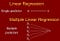Types of Linear Regression

In this blog, I’m going to provide a brief overview of the different types of Linear Regression with their applications to some real-world problems.

# Linear Regression is generally classified into two types:

1. Simple Linear Regression
2. Multiple Linear Regression

# 1. Simple

In Simple Linear Regression, we try to find the relationship between a single independent variable (input) and a corresponding dependent variable (output). This can be expressed in the form of a straight line.

The same equation of a line can be re-written as:## Afroz Chakure

Book lover || Passionate Blogger || GitHub : github.com/afrozchakure || Linkedin : linkedin.com/in/afroz-chakure-489780168